1

## How to Solve the Soma Cube

Published on Thursday, February 27, 2014 in , , , , ,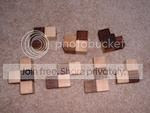"Problems worthy of attack prove their worth by hitting back." - Piet Hein, "Problems"

Besides beings known for his short poems, or “Grooks,” Piet Hein is also the inventor of one of the most classic 3-D puzzles: The Soma Cube.

Even if you're not familiar with the name, you've probably seen it. It's consists of several pieces, each made up of 3-4 cubic units, and the basic challenge is to put these pieces together to form a cube.

It was popularized in the US by no less than Martin Gardner, when he wrote about it in his Sept. 1958 “Mathematical Games” column in Scientific American, and later reprinted in his book, The Second Scientific American Book of Mathematical Puzzles and Diversions. Most of the chapter is excerpted here online.

There are numerous versions, the most commonly available today being ThinkFun's Block by Block version. Puzzles.com features links to several do-it-yourself versions, some requiring little more than tape, strips of paper, and a little time. Some of the links there are no longer functioning, but can still be viewed with help from the Internet Archive's Wayback Machine.

Even if you don't already have the puzzle, and don't want to make one, but still want to play around with it, you can play with it on the computer. The version that caught my eye was one that used Google's free 3-D program Sketchup. Once you learn the basics of rotating and moving objects in Sketchup, and download a free set of Soma Cubes for the program, you're ready for the challenge.

I haven't yet discussed solving the classic Soma Cube challenge, so if you want to solve it for yourself without learning how to solve it, stop reading NOW.

You're still here? OK, then I assume you want to learn how to solve it, and more importantly, how to remember the solution. YouTube user MisterCorzi has posted probably the best Soma Cube solving video I've seen, as it emphasizes the points that make the solution easy to recall:

Once you have the basic idea, here's a diagram of MisterCorzi's approach, for quick and easy reference: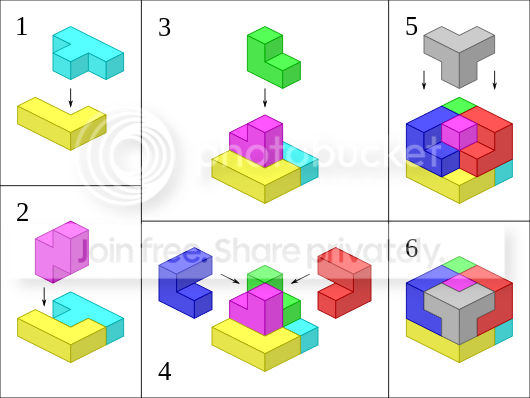I should emphasize that this is only 1 of 240 possible ways (not including rotations or reflections) to solve the Soma Cube, so you can still find plenty of challenge by trying to find the other solutions. There are also many other shapes you can try to make, so create, have fun, and explore!1

## Mental Math: Exponents

Published on Sunday, February 23, 2014 in , , , , ,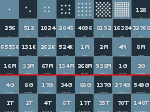There's plenty of mental math shortcuts out there for addition, subtraction, and multiplication. The mental shortcuts for division are harder to find, but they are out there.

When you start asking about mental math shortcuts related to exponents, however, the only methods you find relate to taking roots, like my root extraction tutorial in the Mental Gym. What about actually taking numbers up to various powers instead?

WRAPPING YOUR HEAD AROUND EXPONENTS: Working out exponents seems scary, because most people are familiar with how quickly exponents become large numbers. You've probably heard a version of the classic legend about the reward given to the inventor of chess:

Too many people think of exponents as just repeated multiplying, but it's better to think of it as growth for a given amount of time. BetterExplained.com's article, Understanding Exponents (Why does 0^0 = 1?) is a great place to begin intuitively understanding the nature of exponents.

I've written before about mathematician and engineer Solomon Golomb, who challenged himself to be able to work out, or at least recall, the solution to any problem of the form xy where x and y were any integers (whole numbers) from 1 to 10. In college, a professor mentioned the number 224, joking that "everybody knows what that is." When Solomon Golomb realized that 224 was the same as 88, he was able to immediately reply, "Yes! It's 16,777,216!" The teacher was stunned to learn that it was the correct answer!

I may not get you that far in this post, but I think you'll be surprised just how far you can go with just a little extra mental training.

EASY EXPONENTS: There are a few easy exponents with which you should already be familiar. 1 to any power is always 1. Similarly, 10 to any power is simply 1 followed a number of zeroes equal to the power. 102 is 1 followed by 2 zeroes, or 100, 103 is 1 followed by 3 zeroes, or 1,000, and so son. Also, any number to the first power is always itself.

Squaring, of course, is just multiplying a number by itself. When you learned multiplication starting with times tables, that included multiplying all the numbers from 1 to 10 by themselves, so from this point on, I'll assume you know these already.

CUBES: When a number is raised to the 3rd power, we say it's cubed. A cube is easily worked out by multiplying a number times it's own square. 23 can be determined by multiplying 2 by the 4 (the square of 2) to get 8, and 33 can be determined by multiplying 3 × 9 (the square of 3) to get 27.

After that, we start having to deal with 2-digit numbers by 1-digit numbers. 43 is 4 × 16, and you might be fine working that out in your head, but what about 53 (5 × 25) through 93 (9 × 81)? Fortunately, Mental Math secrets is here to help you learn how to multiply 2-digit numbers by 1-digit numbers:

Once you've mastered this, you can easily work out cubes in your head! Even better, since we're only talking about 10 different cubes, regular practice will make them easy to recall, instead of calculate, so you can get them even quicker.

4TH POWER: Taking a number to the 4th power can be done by squaring the number, and then squaring the result you get. Once again, if you know your times tables, 2 and 3 are easy to take the 4th power. To get 24, we square 2 to get 4, and then square 4 to get 16. For 34, we square 3 to get 9 and then square 9 to get 81.

The problem comes, of course, when we need to square 2 digit number. For 44, it's easy enough to do 4 squared to get 16, but how do we square 16? This problem continues up to 94, for which you need to work out 81 squared. Fortunately, Harvey Mudd College Professor Arthur Benjamin is here to show you how to easily square 2-digit numbers:

Notice what the method of moving to the nearest multiple of 10 does - it turns 2-digit times 2-digit multiplications into 2-digit times 1-digit multiplication, which you learned how to do earlier! In fact, you can use those same techniques to help you work out these problems quickly.

Try this technique and practice working out the numbers 1 through 10 to the 4th power. You'll be amazed how quickly you're able to calculate, and before long just recall, each of these numbers.

5TH POWER: Once you're confident in your ability to work out 4th powers of the numbers in your head, you're ready for 5th powers.

To work out the 5th power of a number, you're going to take that number and multiply it by its own 4th power. 35 is 3 × 34, or 3...9...81, or 243. This is easy, as you've already mastered multiplying 2-digit numbers by 1-digit numbers by this point.

Multiplying numbers with 3 or more digits by a 1-digit number is similar to the process you learned earlier, but you do need to ready to work with more digits. Mental Math Secrets posted this 3-digit by 1-digit multiplication video on their site to help you learn this technique more effectively. Once you get the hang of this, multiplying 4-digit numbers by 1-digit numbers also shouldn't be that difficult.

5th powers also have a neat pattern: Each number 1 through 10, when taken to the 5th power, ends with the same digit as the number with which you started. 25 ends in 2, 35 ends in 3, and so on.

6TH POWER: If you've made it to the point where you can do 5th powers in your head, you may want to stop there. If you're ready for another challenge, however, then you may want to consider learning 6th powers.

To take a number to the 6th power, you'll need to find a number's cube, and then square it. Remember, however, that some of the cubes up to 9 are 3-digit numbers, so this will require the ability to square 3-digit numbers.

Squaring 3-digit numbers was briefly described above in the Arthur Benjamin video, but there are a few points you should know before you practice. First, you should be able to square all the numbers from 1 through 50 as fast as possible. Your adjustments will always be up or down by no more than 50, so this is an essential skill. Second, you'll need to be comfortable multiplying 3-digit numbers by 1-digit numbers.

Finally, when applying Professor Benjamin's technique for squaring 3-digit numbers, there's an easy to way to get that 2nd number you're going to multiply by that multiple of 100. Simply take the last two digits of the number you're squaring and double them, using the hundreds digit plus the final two digits of this doubling (if the number is greater than 100).

For example, when trying to square 729, you're obviously going to multiply by 700, but what's the other number? Simply double 29 (the last 2 digit of 729) to get 58, so you know the other number you need must be 758. What about 343? 43 doubled is 86, so you'll multiply 300 times 386. Once you see the pattern, it's easy to grasp.

Taking the numbers 1 through 10 to the 6th power is as far as I'm going in this post, so you can practice to the level you want. If you find any handy tips or tricks for doing exponents in mental math, I'd love to hear about them in the comments!0

## Fifth Root Feat

Published on Sunday, February 16, 2014 in , , , , , ,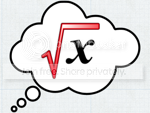It's time to train and strain your brain to entertain!

This time around, we're going to take another look at finding 5th roots. Imagine having someone choose a secret number x, multiplying it by itself a total of 5 times, as in x × x × x × x × x, giving you only the answer, and you're able to work out what the original number x!

It was almost a year ago when Scam School covered how to do cube roots in your head. If you practiced that, you may still be worried about handling 5th roots.

Surprisingly, doing 5th roots in your head is actually easier! Numberphile, with some help from Simon Pampena, explains the process in the following video:

Want to practice this feat for yourself? I have a detailed tutorial on working out 5th roots in your head along with a 5th root quiz designed to help you master this feat. Michael Daniel's Mind Magician site also features a different, but still excellent, 5th root tutorial and quiz, too.

There's little you can do with this other than using it to impress people at parties, but with 5th roots, cube roots, and perhaps even square roots of perfect squares and square roots of non-perfect squares, you'll have plenty of tools in your bag to astound and amuse when the time is right!0

## More Fun With Binomials

Published on Sunday, February 09, 2014 in ,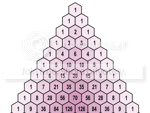I finished the previous post about Prime Test and Pascal's Triangle by mentioning that exploring one idea can often take you to some surprising places.

Exploring those same idea even further, I've run across some additional surprising ideas that I'll share in this post.

• SIMPLIFYING THE TEST: I finished the Pascal's Triangle exploration of the AKS test by mentioning that, if the test returned a row of all zeros, as in this test run on 71, then the given number n is prime.

After seeing this picture, linked from this thread on Reddit, which used the idea of summing (represented by the ∑ symbol) the results of each iteration, I smacked myself in the head for not thinking of that before. Here's the test for 71 re-run with summation. It's certainly much easier to see whether the total is 0 or not.

• FINDING AN ERROR: I thought it might be fun to see how this function looked on a graph, so I headed over to the Desmos.com online graphing calculator. Unfortunately, it didn't work because their software seems to have some sort of bug which occurs when binomials are run through modular arithmetic.

For example, it calculates 462 mod 11 correctly, but when a binomial is calculated as 462, and that binomial is calculated mod 11, then the odd result of 11 occurs (the answer should be 0). I even found another example, where mod returns an answer that isn't even a whole number!

• EASIER PEASIER BINOMIAL EXPANSION: I guess the easiest version of binomial expansion would have to be this picture, but there's a more mathematically correct approach that's also quite easy.

Steven Pomeroy of mathtricks.org teaches a great way of expanding binomial equations, even when Pascal's Triangle isn't handy! If you're interested in just the coefficients, as we were in the AKS primality test, you can even skip down to about half way, where he teaches how to calculate each coefficient in the row.

I've found a way to simplify Mr. Pomeroy's version of the calculation that works better for me, so I'd like to share it with you. Recall that the 0th binomial, the leftmost number on any row of Pascal's Triangle, is always 1, so we always start this with 1.

First, you need a row, so we'll use row 8 as an example. You start by creating a fraction with the row number on top, and a 1 on the bottom. In our 8 example, the first fraction would 81. We'll also be using subsequent fractions in further calculations, which will decrease the numerator (the top number) by 1, while increasing the denominator (the bottom number) by 1. So, the sequence of calculations for row 8 would run as follows:

81, 72, 63, 54, 45, 36, 27, 18

That sequence should be easy enough to understand. Also, note that the last half of that fraction sequence are simply reciprocals of fractions in the first half, so the resulting answer we get when multiplying by these fractions will rise and fall symmetrically, as we discussed in the previous post. So, the only part of the fraction sequence you really need from above are those where the numerator is greater than or equal to (in the case of odd numbered rows) the denominator:

81, 72, 63, 54

After that, the row goes down with the numbers in the same order, due to the symmetry of Pascal's Triangle.

What do we do with these fractions? You're going to multiply them by whole numbers, record the answer, and then use that answer to multiply by the next fraction in the sequence. This may sound confusing, so I'll return to our example using 8.

We always start with 1, and multiply it by the first fraction, which is 81 in this example. 1 × 81 = 8, so we have our first two numbers in the sequence:

1, 8

Next, we adjust the fraction as described above (decrease numerator by 1, increase denominator by 1), and multiply it by our new answer. 8 was our previous answer, and 72 is the next fraction, and 8 × 72 = 28. We already have 3 terms in the sequence:

1, 8, 28

We continue on, each time using the previous answer multiplied by the next fraction in the sequence. 28 × 63 = 56, and 56 × 54 = 70, giving us:

1, 8, 28, 56, 70

Since the next fraction would be a reciprocal of 54, we know that the numbers are going to start decreasing in the same way they increased, so we can just fill out the remaining half symmetrically:

1, 8, 28, 56, 70, 56, 28, 8, 1

Sure enough, that's exactly how the 8th row of Pascal's Triangle appears!

With 9, or any other odd row, you're going to multiply by the following fractions, until you get down to the point where the numerator and denominator are the same:

91, 82, 73, 64, 55

As before, we start with 1, so 1 × 91 = 9, 9 × 82 = 36, 36 × 73 = 84, 84 × 64 = 126, and 126 × 55 = 126, giving us the following sequence of numbers:

1, 9, 36, 84, 126, 126

Obviously, it's time to apply the numbers to the end symmetrically, giving us this sequence, which is how the 9th row of Pascal's Triangle appears:

1, 9, 36, 84, 126, 126, 84, 36, 9, 1

Multiplying by weird fractions like this might seem intimidation, but it's actually easier than it seems. When given ANY fraction, even an improper fraction (one where the numerator, the top number, is greater than the denominator, the bottom number), think of the numerator as the number by which to multiply, and the denominator as the number by which to divide. You can do the multiplication and division needed in either order, as well!

Let's take what is possible the worst example from above, which would be 36 × 73. The numerator is 7 and the denominator is 3, so you could multiply 36 by 7, getting 252, and then dividing that by 3 to get 84. However, in this particular example, it seems easier to divide 36 by 3 first, to get 12, and then multiply that by 7 to get 84. See how easy it is when you know how?

That's all for this post, but that's also plenty to explore, practice, and enjoy!1

## AKS Meets Pascal

Published on Thursday, February 06, 2014 in , , , ,In mathematics, there are many times where you explore an apparently simple idea, and you find unexpected beauty.

Numberphile has recently been posting about tests for prime numbers, also referred to as primality tests, and their latest one fascinated me because of the amazing patterns involved.

Their first video on this topic was about Fermat's primality test, and the fascinating concept of liar numbers. However, I'm going to discuss their more recent video on the AKS primality test, named for the last initials of the authors, Manindra Agrawal, Neeraj Kayal, and Nitin Saxena:

Let's examine this formula a bit closer, with the help of Wolfram|Alpha. The command used to find the expanded form of an equation is simply the command Expand[]. So, if we wanted to find out the expanded form of (x-1)3, we simply enter it as Expand[(x-1)3], and just like in the video, we get the result x3 - 3x2 + 3x - 1.

As in the video, if we subtract x3 - 1, we're left with - 3x2 + 3x, and both coefficients are divisible by the original power, 3, so we know we've got a prime.

Let's try that with the power 4, resulting - 4x3 + 6x2 - 4x + 2. While 4 is obviously evenly divisible by 4, the numbers 6 and 2 are quite obviously not, so 4 isn't prime.

Note that the powers of x don't really matter much, so perhaps it would be nicer to just look at this problem without all the variables and powers getting in the way. Since the AKS test starts with the simple form of (x - 1)p, all the resulting coefficients (the numbers multiplied by the variables and their powers) are simply what are known as binomial coefficients.

Don't worry, binomials are easy to understand when taught properly. They're basically the number of ways k things can be combined of n possibilities without regard to the exact order. BetterExplained.com's post on permutations and combinations makes this clear. What you really want to focus on in that post in the part about combinations, as they are also known as binomials.

Wolfram|Alpha uses the command Binomial[n, k] to work out these combinations, also read as n choose k. For example, entering Binomial[8, 3] gives us 56, telling us that there are 56 ways 3 items can be combined from among 8 possibilities. The Mind Your Decisions blog's fresh fruit puzzle explains how binomials are used in probability.

Now, if we want to see just the coefficients of the cube of (x - 1), we can combine the Binomial[] command with the Table[] command in Wolfram|Alpha to read Table[Binomial[3, k], {k, 0, 3}]. This command is telling Wolfram|Alpha to work out the possible combinations of 0 objects from among 3, then the combinations of 1 object from among 3, then the combinations of 2 objects from among 3, and finally the combinations of 3 objects from among 3, and to put all that information in 1 horizontal table. The result is {1, 3, 3, 1}, which are the same numbers from the calculation above, but without all those messy variables and powers to spoil the view!

Just for fun, let's throw in the Column[] command, and go through the same process for all the numbers from 0 to 10, by entering the command Column[Table[Binomial[n, k], {n, 0, 10}, {k, 0, n}]]. Does that pattern of binomials look familiar?

```.                     {1}
{1, 1}
{1, 2, 1}
{1, 3, 3, 1}
{1, 4, 6, 4, 1}
{1, 5, 10, 10, 5, 1}
{1, 6, 15, 20, 15, 6, 1}
{1, 7, 21, 35, 35, 21, 7, 1}
{1, 8, 28, 56, 70, 56, 28, 8, 1}
{1, 9, 36, 84, 126, 126, 84, 36, 9, 1}
{1, 10, 45, 120, 210, 252, 210, 120, 45, 10, 1}```
If it looks familiar, it's because you probably know it as Pascal's Triangle! I've talked about it before, most often in my annual 12 Days of Christmas post, but also in my Happy 10/11/12! post. I think it's interesting that a test for primes has lead us to Pascal's Triangle!

You might note that all the binomials in Pascal's Triangle are positive, while the multiplication of (x - 1) to various powers gives us alternating positive and negative signs. This is easily remedied, by multiplying every other number in the command above by -1. However, the signs really don't matter in the AKS test, as -35 is just as evenly divisible by 7 as +35, so I'm going to ignore the signs from here on out.

Another thing to notice about the binomials in Pascal's Triangle is that the entire pattern has left/right symmetry. For example, the binomials in the 8th row, representing the coefficients for (x - 1)8, are {1, 8, 28, 56, 70, 56, 28, 8, 1}. The first 5 a different, {1, 8, 28, 56, 70}, but then they start going back down from 70 in the same way they built up. By taking advantage of Wolfram|Alpha's Floor[] command, and only going halfway across each table, we can shorten the list of coefficients we need to examine by half!

Also, remember in the text that for any power p, xp is subtracted, which effectively removes the first coefficient from the list. So, by starting at the 1st coefficient instead of the 0th, we can trim the list even more. Not surprisingly, this eliminates the tables for the power of 0 and 1, so we'll just start the list with coefficients of the power of 2.

At this point, we can look at just the coefficients for any power, without getting the numerous variables and powers in the way, and we've minimized the numbers we need to examine at each step. It's time to run through the AKS primality test again. For each of the numbers 1 through 10, it's not hard to see whether the coefficients are divisible by those numbers, because we're so familiar with them. What about numbers on a larger scale?

Let's turn our Pascal's Triangle generator into a generator for a single power, so we can run tests on individual numbers. We remove the Column[] command, and set n to an individual number. I'm going to use 71 for the first test, since James Grime uses that example at about the 2:20 mark in the video above. The result is this horrendous-looking set: {71, 2485, 57155, 971635, 13019909, 143218999, 1329890705, 10639125640, 74473879480, 461738052776, 2560547383576, 12802736917880, 58104729088840, 240719591939480, 914734449370024, 3201570572795084, 10358022441395860, 31074067324187580, 86680293062207460, 225368761961739396, 547324136192795676, 1243918491347262900, 2650087220696342700, 5300174441392685400, 9964327949818248552, 17629195603524593592, 29381992672540989320, 46171702771135840360, 68461490315822108120, 95846086442150951368, 126764178842844806648, 158455223553556008310, 187265264199657100730, 209296471752557936110, 221256270138418389602} *PHEW!*

Since few of us are familiar with our multiples of 71, you can't easily tell whether all these numbers are evenly divisible by 71. If you could verify that, then this test and our refinements via Pascal's Triangle will verify that 71 is a prime number. If all these numbers were evenly divisible by 71, then there would be no remainder. We can take advantage of this by using modular arithmetic!

If we simply take each of the above numbers, and apply mod 71 to them, we get a far simpler result: {0, 0, 0, 0, 0, 0, 0, 0, 0, 0, 0, 0, 0, 0, 0, 0, 0, 0, 0, 0, 0, 0, 0, 0, 0, 0, 0, 0, 0, 0, 0, 0, 0, 0, 0} Every number is clearly divisible by 71, so 71 is prime!

Using the AKS primality test this way, you can set n to any number, and instantly tell whether than number is prime. Do you see any numbers other than 0? Then it's not a prime number. Is the number 391 prime? If you set n to 391 in this test, you'll see plenty of numbers that aren't 0, so you know 391 isn't prime.

If nothing else, I hope you get an idea of where exploring one idea in math can lead you to some surprising places. Sometimes, it's just fun to explore!3

## Danica McKellar's Math Bites

Published on Sunday, February 02, 2014 in , , , , , , , , ,No, I'm not insulting Danica McKellar's math ability, I swear! I even sell her math books over in the Grey Matters Store!

Math Bites is actually the name of Danica McKellar's new weekly video series from Nerdist, which started last month.

Each episode is only about 5-6 minutes long, and focuses on one math topic. The style is somewhat like that of Square One TV, in that there are short comedy skits and musical segments that help reinforce the concepts or give you breathing time to let the concepts sink in. I especially like the takeaway tips at the end of each episode, which summarize the important points of each episode.

The first episode focused on a topic near and dear to my heart, the constant Pi:

While many Grey Matters readers have memorized Pi to 400 digits, some might prefer the full Dance of the Sugar Pi Fairy to help them memorize Pi to 139 digits.

The second episode talked about the importance of being able to do math in your head. No, this episode doesn't talk about extraordinary mental math feats like those taught in the Mental Gym, but rather knowing how to do basic arithmetic and estimation well enough so that you don't get ripped off:

You can already get an idea of why I like this series so much. It touches on the basics of topics I've posted about many times before. I've posted about binary numbers both on their own, and their importance in Nim, so the 3rd episode on binary numbers was also helpful and entertaining:

The most recent episode as of this posting is about percentages, and focuses on how they can mislead you. Sometimes you can get taken advantage of when businesses can't do math, and also when businesses can do math, so percentages can be very important to understand:

Even if you missed the initial announcement of Math Bites, you're now completely caught up with the series. Here's hoping that Danica McKellar keeps this series up for a long time to come!